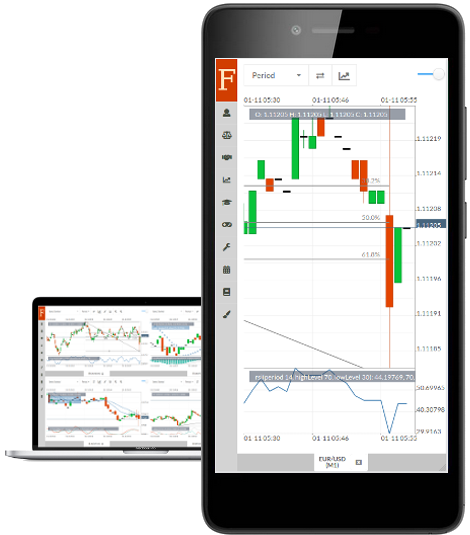# Expert Advisor Studio ~ Genetic Algorithm

The Optimizer of Fintechee expert advisor studio supports optimization for multiple EAs, Genetic algorithm reduces the number of combinations of test cases.

## # Optimizer

Fintechee optimizer supports optimization for multiple expert advisors, Genetic algorithm for trading helps traders reduce the number of combinations.

The old version of Fintechee can just optimize one EA's parameters. Now Fintechee supports a feature to optimize for several EAs simultaneously. This feature is very useful for backtesting a portfolio by optimizing several EAs at the same time. But it brings us more parameters to optimize and makes it more difficult to find out a better combination of parameters. We need to take more time to optimize as well.

I often use three moving average indicators alongside to monitor the short-term and long-term market movements. Meantime, I use RSI, MACD, ADX, Stochastic Oscillators as well. They are very common technical indicators, I think more than 90% of traders are using them. But I think nobody can convince me which values for the "period" parameters of the indicators work. So I need to try every possible value to test. I code expert advisors, so I need to backtest all the combinations as well. It's a mission impossible to backtest them under the traditional optimizer.

## # Genetic Algorithm

Now, we can achieve it. Because Fintechee released a very useful feature: a Genetic Algorithm. Please refer to other articles to know more details about the theory. We won't discuss the theory here, we will just show you how to apply this theory on trading and help us optimize our trading strategies faster.

If you follow our posts here, you must have read my previous posts about Artifact Intelligence for trading, such as Neural Network. The neural network model can be integrated with the genetic algorithm as well.

For example, we can build a neural network, and train the model's parameters(such as the weight, the bias) by the so-called Gradient Descent. But we all know it may fall into local optimum. The genetic algorithm can train the parameters by global optimum. So we can apply this advantage to the neural network.

In Fintechee WEB trader, we have integrated a third-party neural network Library: Synaptics, so you are not required to use the genetic algorithm to optimize the weights and the biases that this Library generates. You can build a neural network from scratch on to see how it works by integrating a genetic algorithm with it.

Here I have created an example:

``````registerEA(
"nn_example",
"A test EA to run neuron model for XOR(v1.0)",
[
// hidden layer(1st neuron)
{name: "h11", value: 20, required: true, type: "Number", range: [-100, 100], step: 10},
{name: "h12", value: 20, required: true, type: "Number", range: [-100, 100], step: 10},
{name: "b1", value: -10, required: true, type: "Number", range: [-100, 100], step: 10},
// hidden layer(2nd neuron)
{name: "h21", value: -20, required: true, type: "Number", range: [-100, 100], step: 10},
{name: "h22", value: -20, required: true, type: "Number", range: [-100, 100], step: 10},
{name: "b2", value: 30, required: true, type: "Number", range: [-100, 100], step: 10},
// output layer
{name: "o1", value: 20, required: true, type: "Number", range: [-100, 100], step: 10},
{name: "o2", value: 20, required: true, type: "Number", range: [-100, 100], step: 10},
{name: "b", value: -30, required: true, type: "Number", range: [-100, 100], step: 10},
],
function (context) { // Init()
// Please don;t remove the source codes below, they are required to make an EA get boosted
var account = getAccount(context, 0)
var brokerName = getBrokerNameOfAccount(account)
var accountId = getAccountIdOfAccount(account)
var symbolName = "EUR/USD"

getQuotes (context, brokerName, accountId, symbolName)

},
function (context) { // Deinit()
var h11 = getEAParameter(context, "h11") // weight
var h12 = getEAParameter(context, "h12") // weight
var b1 = getEAParameter(context, "b1") // bias
var h21 = getEAParameter(context, "h21")
var h22 = getEAParameter(context, "h22")
var b2 = getEAParameter(context, "b2")
var o1 = getEAParameter(context, "o1") // weight
var o2 = getEAParameter(context, "o2") // weight
var b = getEAParameter(context, "b") // bias

var sigmoid = function (t) {
return 1 / (1 + Math.pow(Math.E, -t))
}

var nn = function (p1, p2) {
return sigmoid(o1 * sigmoid(h11 * p1 + h12 * p2 + b1) + o2 * sigmoid(h21 * p1 + h22 * p2 + b2) + b)
}

var error = 0

error += nn(1, 1)
error += 1 - nn(1, 0)
error += 1 - nn(0, 1)
error += nn(0, 0)

setOptimizationResult(context, -error)

printMessage("error: " + error + ", " + Math.round(nn(1, 1)) + " " + Math.round(nn(1, 0)) + " " + Math.round(nn(0, 1)) + " " + Math.round(nn(0, 0)))
},
function (context) { // OnTick()
})

``````

This neural network model includes 2 layers, the hidden layer includes 2 neurons, the output layer includes 1 neuron. I just made an example, so I coded it as simple as possible.

I use setOptimizationResult API to set the value for the fitness function built-in inside of Fintechee. The fitness function will monitor the parameters of the neural network to know when to stop training.

If you are already familiar with Fintechee SDK, you may find we have a new property, called "step". It will set the step between the start value and the end value for the optimization. If you leave this value by null, then the system will make the difference between the start value and the end value as the step value.